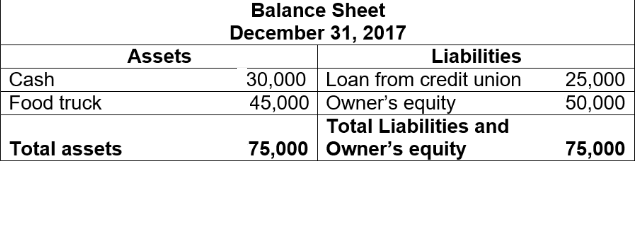# Accounting Equation

Accounting equation is referred to as the relationship between assets, liabilities and capital of a business. The accounting equation is one of the most important equations in accounting and is used for preparing balance sheet. It can be represented by the following equation:

A = C + L

where ,

A = Assets

C  = Capital (Owner’s equity)

L  = Liabilities

The accounting equation can be also be represented as :

Assets = Liabilities + Capital (Owner’s equity)

This equation can be used to find the value of Capital and Liabilities

Capital =  Assets – Liabilities

Or

Liabilities = Assets – Capital

The accounting equation sometimes is also referred to as the balance sheet equation since the accounting equation shows the fundamental relationship between the assets, liabilities and capital which are regarded as the most components of a balance sheet.

The accounting equation states that at any given point in time, the resources of the business entity (assets) must be equal to the claims of those who have provided finance for those resources.

The claims can be either from proprietors (known as capital) and from outsiders (known as liabilities).

The accounting equation is all about the equality of the assets and liabilities side with each other.

## Key Points of Balance Sheet Equation:

Though Balance sheet equation gives the investors and shareholders a rough idea about the financial status of the company. Few things that should be kept in mind are stated below:

• The report of the Balance Sheet does not always give the true and exact status of the company’s finance
• Each transaction recorded in the financial statements should be mentioned in the Balance sheet
• If one aspect of the Balance sheet is impacted the other aspect should also be influenced

Related link: Balance Sheet vs Cash Flow Statement

### Example:

ABC starts a food truck business. He puts ₹ 50,000 as a capital fund. He further loans ₹ 25,000 from a local credit vendor. Now, he has a total of ₹ 75,000, he then purchases a fully furnished truck for ₹ 45,000.

Below is the ABC balance sheet for December 2017.In the above example, the total assets are equivalent to the total liabilities + owner’s equity.

This concludes the article on the topic of Accounting Equation or Balance Sheet Equation, which is an important topic for Commerce students. For more such interesting articles, stay tuned to BYJU’S.

 Important Topics in Accountancy: